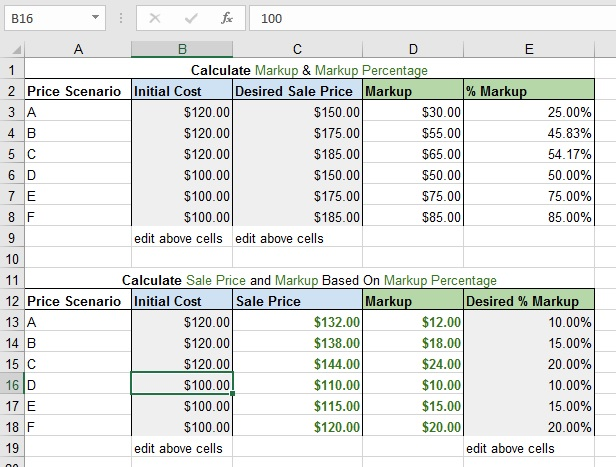# Markup Calculator

I developed a spreadsheet to quickly calculate the markup and markup percentage based on the desired sale price. Also, the spreadsheet is able to calculate the markup and sale price based on the desired percentage markup. It is extremely useful for price variance analysis.Only the grey background cells should be edited. Simply enter the initial cost of the item and the desired sale price to calculate the markup/markup percentage of the product or service. If you want to know the sale price of the item/service, use the bottom calculator. Enter the initial cost of the item and the desired markup percentage (profit margin). The spreadsheet will quickly calculate the sale price and the markup (profit) you will receive. This is very beneficial when providing a quick quote. The numbers above are for demonstrational purposes. The formulas for the cells are available by opening the spreadsheet in Microsoft Excel.

Connect